• +91 9971497814
• info@interviewmaterial.com

# RD Chapter 4- Algebraic Identities Ex-MCQS Interview Questions Answers

### Related Subjects

Question 1 :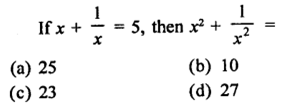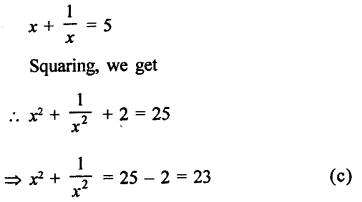Question 2 :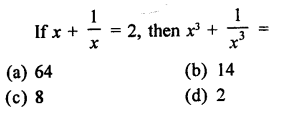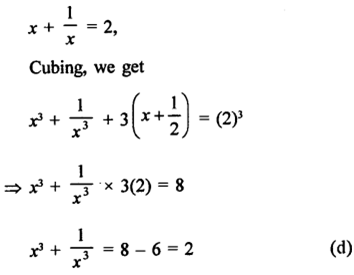Question 3 :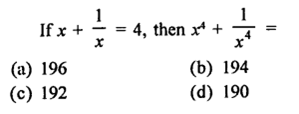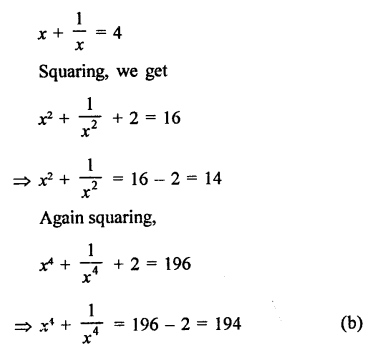Question 4 :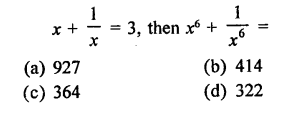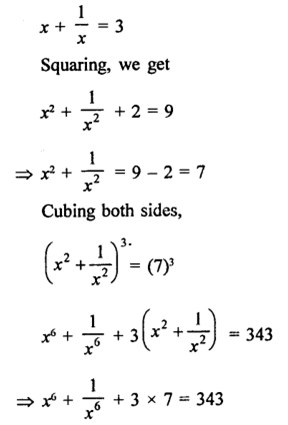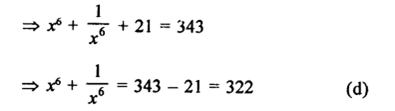Question 5 :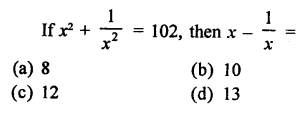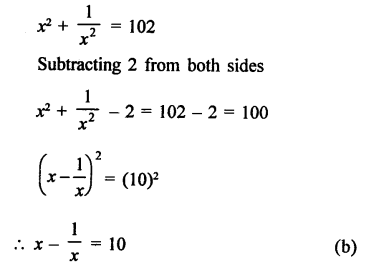Question 6 :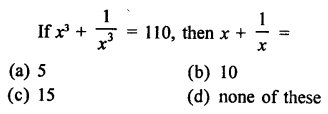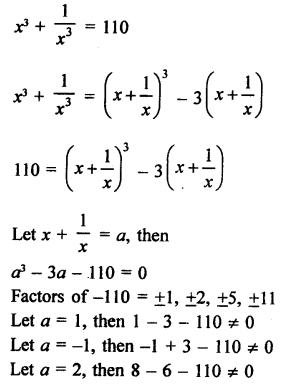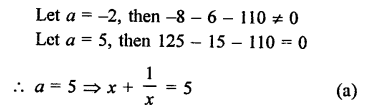Question 7 :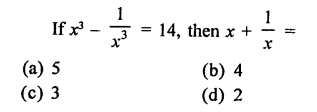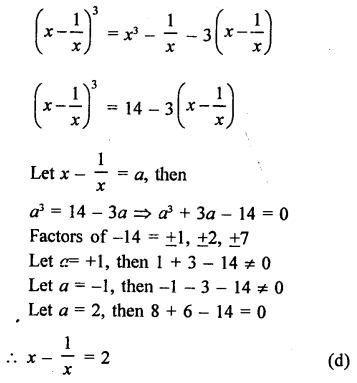Question 8 :
If a + b + c = 9 and  ab + bc + ca = 23, then a2 + b2 + c2 =
(a) 35
(b) 58
(c) 127
(d) none of these

a + b + c = 9, ab + bc + ca = 23
Squaring,
(a + b+ c) = (9)2
a2 + b2 + c2 + 2 (ab + bc+ ca) = 81
a2 +b2 + c2 + 2 x 23 = 81
a2 + b2+ c2 + 46 = 81
a2 + b2+ c2 = 81 – 46 = 35  (a)

Question 9 : (a – b)3 + (b – c)3 +(c – a)3 =
(a) (a + b + c) (a2 + b2 + c2 –ab – bc – ca)
(d) (a -b)(b- c) (c – a)
(c) 3(a – b) (b – c) (c – a)
(d) none of these

(a – b)3 + (b- c)3 + (c- a)3
a – b+ b – c + c – a = 0
(a – b)3 +(b – c)3 +(c – a)3
= 3
(a-b)(b- c) (c – a)        (c)

Question 10 :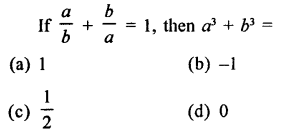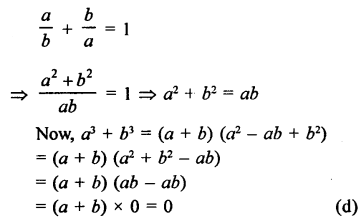Todays Deals### RD Chapter 4- Algebraic Identities Ex-MCQS Contributorskrishan

Name:
Email:

# Latest News# 9000 interview questions in different categories